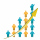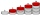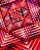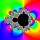# Insert

Insert five numbers between 8 and 27 such numbers that, with two given ones, they form the first seven members of the geometric sequence.

a2 =  9.798
a3 =  12
a4 =  14.6969
a5 =  18
a6 =  22.0454

### Step-by-step explanation:Did you find an error or inaccuracy? Feel free to write us. Thank you!## Related math problems and questions:

• Two geometric progressionsInsert several numbers between numbers 6 and 384 so that they form with the given GP numbers and that the following applies: a) the sum of all numbers is 510 And for another GP to apply: b) the sum of entered numbers is -132 (These are two different geome
• Geometric progressionIn geometric progression, a1 = 7, q = 5. Find the condition for n to sum first n members is sn≤217.
• Sequence - 5 membersWrite first five members of the sequence ?
• Geometric sequenceIn the geometric sequence is a4 = 20 a9 = -160. Calculate the first member a1 and quotient q.
• Geometric sequence 3In geometric sequence is a8 = 312500; a11= 39062500; sn=1953124. Calculate the first item a1, quotient q, and n - number of members by their sum s_n.
• Insert 3Insert five arithmetic progression members between -7 and 3/2.
• Geometric sequence 4It is given geometric sequence a3 = 7 and a12 = 3. Calculate s23 (= sum of the first 23 members of the sequence).
• Insert into GPBetween numbers 5 and 640, insert as many numbers to form geometric progression so the sum of the numbers you entered will be 630. How many numbers must you insert?
• SequenceBetween numbers 1 and 53 insert n members of the arithmetic sequence that its sum is 702.
• Five elementThe geometric sequence is given by quotient q = 1/2 and the sum of the first six members S6 = 63. Find the fifth element a5.
• GP - three membersThe second and third of a geometric progression are 24 and 12(c+1), respectively, given that the sum of the first three terms of progression is 76. determine the value of c.
• Exponential equationFind x, if 625 ^ x = 5 The equation is exponential because the unknown is in the exponential power of 625
• SequenceCalculate what member of the sequence specified by (5n-2+15) has value 20.
• Sequences AP + GPThe three numbers that make up the arithmetic sequence have the sum of 30. If we subtract from the first 5, from the second 4 and keep the third, we get the geometric sequence. Find AP and GP members.
• Insert 7Insert five harmonic means between 3 and 18
• GP membersThe geometric sequence has 10 members. The last two members are 2 and -1. Which member is -1/16?
• Geometric progressionFill 4 numbers between 4 and -12500 to form geometric progression.